### IMO Shortlist 2010 problem N4

Kvaliteta:
Avg: 0,0
Težina:
Avg: 7,0
Let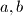$a, b$ be integers, and let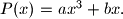$P(x) = ax^3+bx.$ For any positive integer$n$ we say that the pair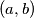$(a,b)$ is$n$-good if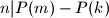$n | P(m)-P(k)$ implies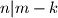$n | m - k$ for all integers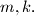$m, k.$ We say that$(a,b)$ is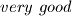$very \ good$ if$(a,b)$ is$n$-good for infinitely many positive integers$n.$
(a) Find a pair$(a,b)$ which is 51-good, but not very good.(b) Show that all 2010-good pairs are very good.
Proposed by Okan Tekman, Turkey
Izvor: Međunarodna matematička olimpijada, shortlist 2010